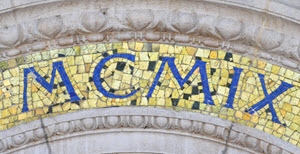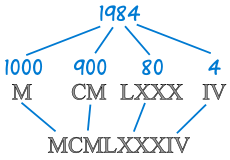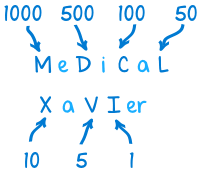# Roman NumeralsAncient Romans used a special method of showing numbers

Examples: They wrote V instead of 5
And wrote IX instead of 9

Read on to learn about Roman Numerals or go straight to the Roman Numeral Conversion Tool.

## The Roman Symbols

Romans Numerals are based on the following symbols:

 1 5 10 50 100 500 1000 I V X L C D M

## Basic Combinations

Which can be combined like this:

 1 2 3 4 5 6 7 8 9 I II III IV V VI VII VIII IX 10 20 30 40 50 60 70 80 90 X XX XXX XL L LX LXX LXXX XC 100 200 300 400 500 600 700 800 900 C CC CCC CD D DC DCC DCCC CM

## Forming Numbers - The Rules

When a symbol appears after a larger (or equal) symbol it is added

• Example: VI = V + I = 5 + 1 = 6
• Example: LXX = L + X + X = 50 + 10 + 10 = 70

But if the symbol appears before a larger symbol it is subtracted

• Example: IV = V − I = 5 − 1 = 4
• Example: IX = X − I = 10 − 1 = 9

To Remember: After Larger is Added

Don't use the same symbol more than three times in a row (but IIII is sometimes used for 4, particularly on clocks)

## How to Convert to Roman Numerals

Break the number into Thousands, Hundreds, Tens and Ones, and write down each in turn.

### Example: Convert 1984 to Roman Numerals.Break 1984 into 1000, 900, 80 and 4, then do each conversion

• 1000 = M
• 900 = CM
• 80 = LXXX
• 4 = IV

1000 + 900 + 80 + 4 = 1984, so 1984 = MCMLXXXIV## How To Remember

Think "MeDiCaL XaVIer".
It has the roman numerals in descending order from 1000 to 1.

I, for one, like Roman numerals!

## Really Big Numbers

Numbers greater than 1,000 are formed by placing a dash over the symbol, meaning "times 1,000", but these are not commonly used:

 5,000 10,000 50,000 100,000 500,000 1,000,000 V X L C D M

## Conversion Tool

You can convert to/from Roman Numerals here: